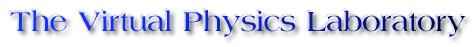Glossary

 Index  |  Atomic Physics  |  Mechanics  |  Optics  |  Waves

angle of incidence
The angle between a ray which is incident on a surface, and the normal to that surface.

angle of reflection
The angle between a ray which is reflected from a surface, and the normal to that surface.

angle of refraction
The angle between the ray which is refracted at a surface and the normal to that surface.

angular frequency
Angular frequency is typically denoted by the Greek letter omega. Typical units: radians/second. omega = 2*PI*f, where f denotes frequency

birefringence
A material is birefringent if the index of refraction differs for incident electromagnetic waves with different polarizations. Examples of birefringent media include calcite (CaCO3), quartz (SiO2), and ice (H2O).

Brewster's angle
An electromagnetic wave, incident on a surface can be broken down into two polarization states. One in which the electric field is polarized perpendicular to the plane of incidence, and another which is polarized parallel to the plane of incidence. Brewster's angle is the angle of incidence at which only the component of the electric field polarized normal to the incident plane is reflected. Hence, if unpolarized light is reflected of a surface at Brewster's angle, the reflected light is completely polarized. Brewster's angle occurs when the angle between the reflected and refracted rays are 90 degrees. Named for Sir David Brewster who discovered it empirically.

diffraction
Diffraction, the deviation of light from rectilinear propagation, is a characteristic of wave phenomena which occurs when a portion of a wave front is obstructed in some way. When various portions of a wave front propagate past some obstacle, and interfere at a later point past the obstacle, the pattern formed is called a diffraction pattern.

dispersion
The dependence of the velocity of a wave on the frequency of the wave is known as dispersion. A media in which waves of different frequencies propagate at different speeds is said to be dispersive.

Fermat's principle
Fermat's principle of least time states that in traveling from point A to point B light follows the path which takes the least time.

frequency
The number of oscillations or vibrations per unit time. SI units Hz (Hertz). Frequency is the he inverse of the period T.

group velocity
The speed of the modulated signal. vg = dw/dk

Huygens' Principle
Each point on a propagating wave can be considered as the source of a small wave, or wavelet which propagates at the same speed as the original wave. The new wave front for the propagating wave can be constructed by drawing a line tangent to the edges of these wavelets.

The intensity of a light wave, commonly denoted by the letter I. The irradiance is the power per unit area carried by an electromagnetic wave. The time averaged value of the magnitude of the Poynting vector.

index of refraction
The index of refraction is the ratio of the speed an electromagnetic wave in vacuum to the speed of the electromagnetic wave in a particular medium. typically denoted by the lowercase n. n = c/v. The index of refraction is a property of the medium in question, and the incident electromagnetic wave. In general, they index of refraction may depend on on the frequency of the incident wave, and on its polarization. A media in which the index of refraction depends on the frequency of the electromagnetic wave is said to be dispersive. A media in which the index of refraction depends on the polarization of the incident wave is said to be birefringent

monochromatic
Light of a single wavelength.

period
The time to complete a cycle.

phase constant
The constant phi in a simple harmonic wave: A(x,t) = Am sin(kx-wt+phi).

phase velocity
The velocity of a carrier wave in a modulated signal.

Poynting Vector
Commonly denoted by the letter S. S = (1/mu0) E x B. The Poynting vector represents the flux of energy density per unit time through a unit area. The time averaged value of the Poynting vector is the irradiance. Named after John Henry Poynting

plane of incidence
The plane defined by the incident, reflected and refracted rays. These rays lie in a plane known as the plane of incidence.

simple harmonic wave
Also known as harmonic waves. Any wave form can be broken down into a combination of simpler waves which are sinusoidal. These sines and cosines are known as simple harmonic waves.

Mach number
The ratio of the speed of an object to the speed of sound.

wavelength
The distance between to successive peaks of a periodic wave. Wavelength is typically denoted by the Greek letter lambda.

wavenumber
Typically denoted by the letter k. k = 2*PI/lambda, in a simple harmonic wave: A(x,t) = Am sin(kx-wt+phi).

 Department of Physics and Astronomy - Northwestern University